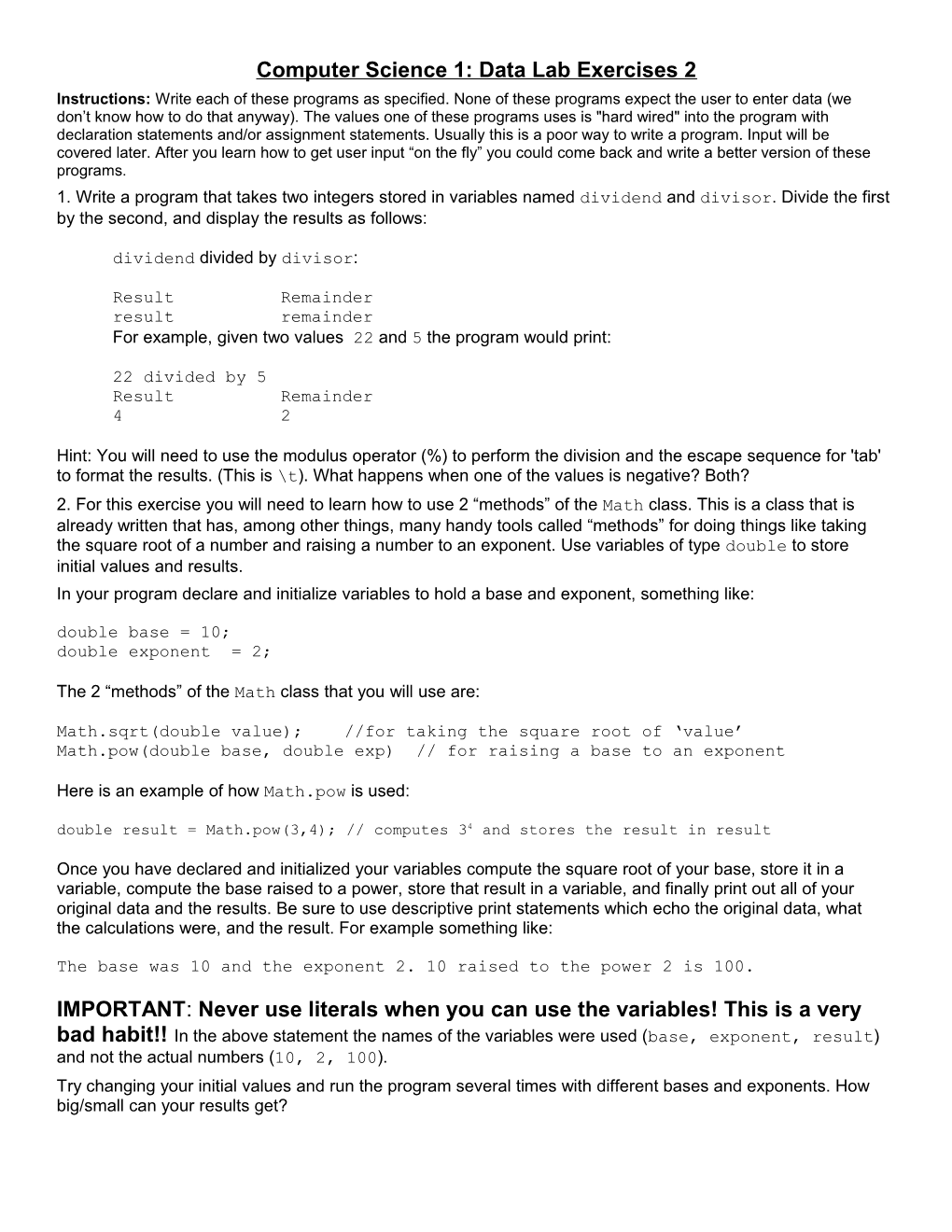# Computer Science 1: Chapter 2 Lab ExercisesComputer Science 1: Data Lab Exercises 2

Instructions: Write each of these programs as specified. None of these programs expect the user to enter data (we don’t know how to do that anyway). The values one of these programs uses is "hard wired" into the program with declaration statements and/or assignment statements. Usually this is a poor way to write a program. Input will be covered later. After you learn how to get user input “on the fly” you could come back and write a better version of these programs.

1.Write a program that takes two integers stored in variables named dividend and divisor. Divide the first by the second, and display the results as follows:

dividend divided by divisor:

ResultRemainder

resultremainder

For example, given twovalues 22 and 5 the program would print:

22 divided by 5

ResultRemainder

42

Hint: You will need to use the modulus operator (%) to perform the division and the escape sequence for 'tab' to format the results. (This is \t). What happens when one of the values is negative? Both?

2. For this exercise you will need to learn how to use 2 “methods” of the Math class. This is a class that is already written that has, among other things, many handy tools called “methods” for doing things like taking the square root of a number and raising a number to an exponent. Use variables of type double to store initial values and results.

In your program declare and initialize variables to hold a base and exponent, something like:

double base = 10;

double exponent = 2;

The 2 “methods” of the Math class that you will use are:

Math.sqrt(double value); //for taking the square root of ‘value’

Math.pow(double base, double exp) // for raising a base to an exponent

Here is an example of how Math.pow is used:

double result = Math.pow(3,4); // computes 34 and stores the result in result

Once you have declared and initialized your variables compute the square root of your base, store it in a variable, compute the base raised to a power, store that result in a variable, and finally print out all of your original data and the results. Be sure to use descriptive print statements which echo the original data, what the calculations were, and the result. For example something like:

The base was 10 and the exponent 2. 10 raised to the power 2 is 100.

IMPORTANT: Never use literals when you can use the variables! This is a very bad habit!!In the above statement the names of the variables were used (base, exponent, result) and not the actual numbers (10, 2, 100).

Try changing your initial values and run the program several times with different bases and exponents. How big/small can your results get?